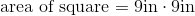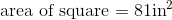ISEE Middle Level Math : Quadrilaterals

Example Questions

Example Question #31 : Squares

What is the area of a square if the length of one side is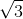?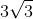Explanation:

The area of a square is found by multiplying one side by itself.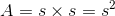We are given the side length, allowing us to solve.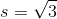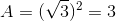Example Question #32 : Squares

A square has an area of 36, and each side is equal toWhat is the value of?Explanation:

Each side of a square is equal to the square root of the area. The square root of 36 is 6, so each side is 6. Thus, 3x (being a side of the square is equal to 6. This means that x is equal to 2 because 3 times 2 is 6.

Example Question #33 : Squares

Find the area of a square with side length 10.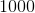Explanation:

To find the area of a square, simply use the formula. Thus,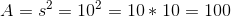Example Question #34 : Squares

The area of a square is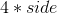?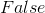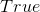Explanation:

The area of a square is the side length squared not the side length times.

Example Question #35 : Squares

If you are given one side length of a square, you can find the area with that information.

True

False

True

Explanation:

To find the area of a square, you multiple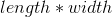.  But with a square all the sides are equal so the equation really is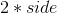or the side length squared.  Since you are given the side length, you can find the area.

Example Question #36 : Squares

A new building in New York City will have a footprint that is a perfect square, taking up a complete city block. If one city block is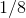of a mile, what is the area taken up by the building?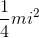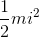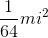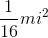Explanation:

A new building in New York City will have a footprint that is a perfect square, taking up a complete city block. If one city block isof a mile, what is the area taken up by the building?

Find the area of a square by raising the side length to the second power: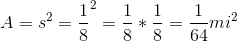So our area is:Example Question #37 : Squares

Use the following to answer the question.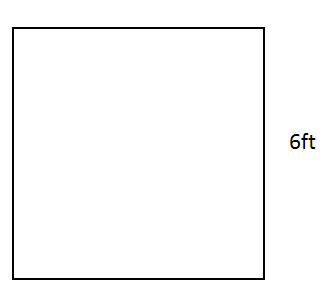Find the area of the square.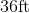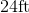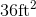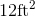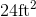Explanation:

When finding the area of a square, we use the following formula: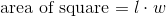where l is the length and w is the width of the square.

Now, we know that a square has 4 equal sides.  So given the squarewe know the length is 6ft and the width is 6ft.  So, we can substitute into the formula.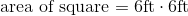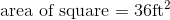Note that the label is also squared.  This is because when we multiply, we are also multiplying the label.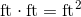Example Question #38 : Squares

Find the area of a square with a width of 8 inches.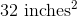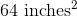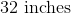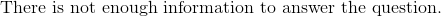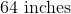Explanation:

To find the perimeter of a square, we use the following formula:where l is the length and is the width of the square.

Now, we know the width of the square is 8 inches.  Because it is a square, we know that all sides are equal.  Therefore, the length is also 8 inches.  Knowing this, we can substitute into the formula.  We get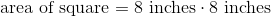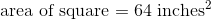Example Question #39 : Squares

You are playing Monopoly and notice that the dice is a cube. If the dice has a height of 2 centimeters, what is the area of one of its surfaces?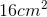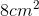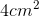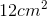Explanation:

You are playing Monopoly and notice that the dice is a cube. If the dice has a height of 2 centimeters, what is the area of one of its surfaces?

We can begin by realizing that we need to find the area of just one surface of the cube. This surface will be a square, we know this because we are dealing with a cube.

So, we need to find the area of a square. Easy enough with the following formula: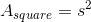Now, we just need to know "s." To find s, we need to look at the question. We are told that the height of the cube is 2cm. This means that the side length of our base (square) is also 2.

So...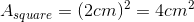Example Question #40 : Squares

Find the area of a square with a base of 9 inches.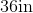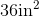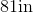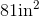Explanation:

To find the area of a square, we will use the following formula:where l is the length and w is the width of the square.

Now, we know the square has a base of length 9 inches.  Because it is a square, we know that all sides are equal.  This means all sides are 9 inches.  Therefore, the width is also 9 inches.  Knowing this, we can substitute into the formula.  So,